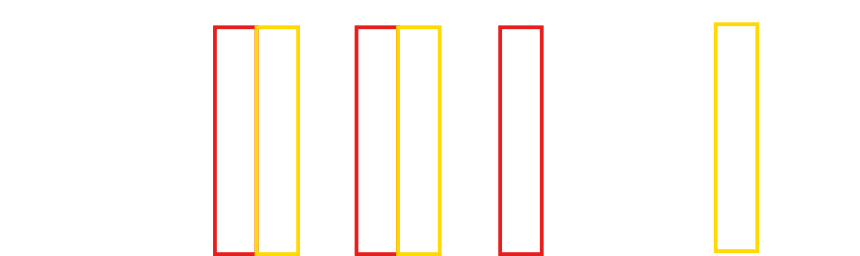# Batch Normalization and ResNets

You have now learned enough to create basic convolutional neural networks that can be used for object classification. Before moving on to the next section about object detection algorithms, there are some other techniques that are commonly used in neural networks that are important to know: batch normalization and residual blocks.

## Batch Normalization

Batch normalization is a technique that is used to speed up training and provides some regularization . The idea behind batch normalization is to try to tackle a problem called the internal covariate shift problem. This problem arises when using training a layer deep in a neural network. When updating the weights of the layers, the model assumes that the weights in the earlier layers are fixed. Updating those earlier layers would likely change the distribution of the input into the next layer which then has an effect on the next layer and so forth. Therefore, updating the weights of a layer deep in the neural network is like chasing a moving target, making these weights more difficult to converge.

\begin{equation} \tag{3.1} \hat{X_i} = \frac{X_i-\mu_B}{\sigma_B + \epsilon} \end{equation}

\begin{equation} \tag{3.2} y = \gamma \hat{X_i} + \beta \end{equation}

Batch normalization tackles the covariate shift problem by standardizing the input (Xi) going into the layer for each mini-batch when training with mini-batch gradient descent. Standardization means calculating the mini-batch’s mean (μB) and standard deviation (σB) and then setting them to be $0$ and $1$ respectively (Eq. 3.1). The inputs are then scaled and shifted by $\gamma$ and $\beta$ which are variables that can be trained (Eq. 3.2). After training the model, the mean and standard deviation can be set to be the ones observed in the training dataset.

In summary, batch normalization is used to speed up convergence in training. Additionally, though it is not considered its primary purpose, batch normalization offers some regularization effect.

## Residual Networks

Generally, the deeper a neural network is, the more complex features or functions it can create, and the more accurate the network can be. So, there is often a preference to create deeper and deeper networks with the drawback of having longer training and computational times. Deep neural networks that are dozens of layers deep can run into a problem called the vanishing gradients problem.

To understand the vanishing gradients problem, it is helpful to understand that the backpropagation step for neural networks involves the repeated use of the chain rule to find the gradient of each layer starting from the last layer. This means that the gradients of the earlier layers depend on the gradients of each successive layer. If, however, the average gradient is $0.9$ with respect to the next layer, for example, the gradient for the first layer would be ~$(0.9)^n$ with respect to the output where $n$ is the number of layers. This causes the gradient to be very small, hence the vanishing gradient problem. These very small gradients are used to update the layers’ weights, causing problems when training the network. Similarly, there is also an exploding gradient problem when the average gradient is greater than $1$, but that problem is much less common.Figure 18: Example of a residual block. The ReLU activation functions are explicitly shown here.

Residual networks use skip connections or shortcuts to mitigate the vanishing gradient problem . They work by using shortcut or skip connections that carry the input and adding it to the output a couple of layers deeper, skipping past several layers. These shortcut connections mean organizing the network model into residual blocks where a block represents the layers between a shortcut connection (see figure 18). When completing the backpropagation step, the shortcut connections can carry the gradient past several layers by reducing the number of layers the gradient has to propagate through; thus mitigating the vanishing/exploding gradient problem.

Normally, many of these residual blocks will be used in a neural network one after the other. It is fairly common to encounter the use of these residual blocks in very deep neural networks such as ones used in YOLOv3 and some versions of Faster R-CNN including the one used to create the video on this website’s homepage.

   Ioffe, S., & Szegedy, C. (2015). Batch Normalization: Accelerating Deep Network Training by Reducing Internal Covariate Shift. ArXiv:1502.03167 [Cs]. http://arxiv.org/abs/1502.03167

   Szegedy, C., Toshev, A., Erhan, D. (2013). Deep Neural Networks for Object Detection. NeurIPS 2013. https://papers.nips.cc/paper/2013/file/f7cade80b7cc92b991cf4d2806d6bd78-Paper.pdf